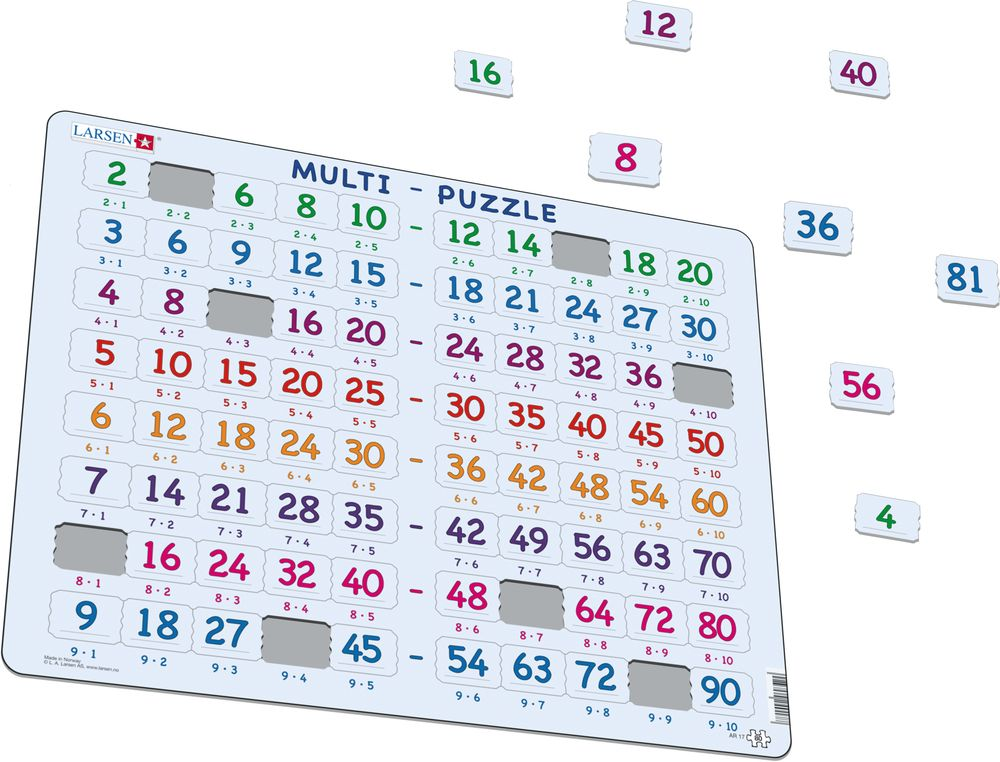# AR17 - Multi-Puzzle

## Maxi (36.5x28.5 cm) with 80 pieces

•Learn the multiplication table. There is the answer to an arithmetic problem on each piece. First you multiply by two (the green row), then by three (the blue row) and so on. Place the answers by their correct arithmetic problem. The pieces will only fit in their proper place.

AR17 - Multi-Puzzle is available in the following versions:

• Neutral (EAN/GTIN: 7023852117507)